# The STDRATE Procedure

### Attributable Fraction and Population Attributable Fraction

Subsections:

The attributable fraction measures the excess event rate or risk fraction in the exposed population that is attributable to the exposure. That is, it is the proportion of event rate or risk in the exposed population that would be reduced if the exposure were not present. In contrast, the population attributable fraction measures the excess event rate or risk fraction in the total population that is attributable to the exposure.

In the STDRATE procedure, you can compute the attributable fraction by using either indirect standardization or Mantel-Haenszel estimation.

#### Indirect Standardization

With indirect standardization, you specify a study population that consists of subjects who are exposed to a factor, such as smoking, and a reference population that consists of subjects who are not exposed to the factor. Denote the numbers of events in the study and reference populations by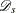and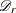, respectively.

For the rate estimate, denote the population-times in the study and reference populations by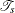and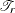, respectively. Then the event rates in the two populations can be expressed as the following equations, respectively: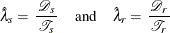Similarly, for the risk estimate, denote the numbers of observations in the study and reference populations by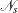and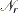, respectively. Then the event risks in the two populations can be expressed as the following equations, respectively: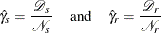In the next two subsections,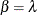denotes the rate statistic and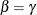denotes the risk statistic.

#### Attributable Fraction with Indirect Standardization

The attributable fraction is the fraction of event rate or risk in the exposed population that is attributable to exposure: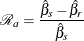With a standardized mortality ratio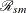, the attributable fraction is estimated by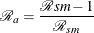The confidence intervals for the attributable fraction can be computed using the confidence intervals for. That is, with a confidence interval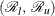for, the corresponding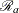confidence interval is given by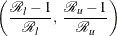#### Population Attributable Fraction with Indirect Standardization

The population attributable fraction for a population is the fraction of event rate or risk in a given time period that is attributable to exposure. The population attributable fraction is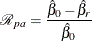where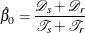is the combined rate in the total population for the rate statistic and where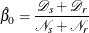is the combined risk in the total population for the risk statistic.

Denote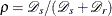, the proportion of exposure among events, then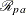can also be expressed as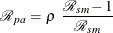whereis the standardized mortality ratio.

An approximate confidence interval for the population attributable ratecan be derived by using the complementary log transformation (Greenland 2008, p. 296). That is, with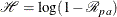a variance estimator for the estimated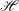is given by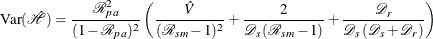where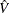is a variance estimate for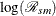.

#### Mantel-Haenszel Estimation

With Mantel-Haenszel estimation, you specify one study population that consists of subjects who are exposed to a factor and another study population that consists of subjects who are not exposed to the factor. Denote the numbers of events in the exposed and nonexposed study populations by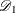and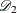, respectively.

For the rate estimate, denote the population-times in the two populations by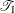and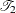, respectively. Then the event rates in the two populations can be expressed as the following equations, respectively: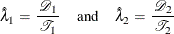Similarly, for the risk estimate, denote the numbers of observations in the two populations by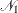and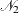, respectively. Then the event risks in the two populations can be expressed as the following equations, respectively: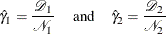In the next two subsections,denotes the rate statistic anddenotes the risk statistic.

#### Attributable Fraction with Mantel-Haenszel Estimation

The attributable fraction is the fraction of event rate or risk in the exposed population that is attributable to exposure: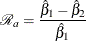Denote the rate or risk ratio by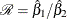. The attributable fraction is given by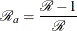The confidence intervals for the attributable fraction can be computed using the confidence intervals for the rate or risk ratio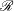. That is, with a confidence intervalfor, the correspondingconfidence interval is given byFor Mantel-Haenszel estimation, you can use the Mantel-Haenszel rate or risk ratio to estimate.

#### Population Attributable Fraction with Mantel-Haenszel Estimation

The population attributable fraction for a population is the fraction of event rate or risk in a given time period that is attributable to exposure. The population attributable fraction is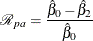where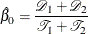is the combined rate in the total population for the rate statistic and where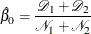is the combined risk in the total population for the risk statistic.

Denote the proportion of exposure among events as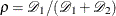. Thencan also be expressed as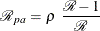whereis the rate or risk ratio.

An approximate confidence interval for the population attributable ratecan be derived by using the complementary log transformation (Greenland 2008, p. 296). That is, witha variance estimator for the estimatedis given by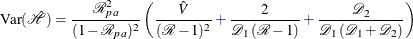whereis a variance estimate for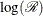.

For Mantel-Haenszel estimation, you can use the Mantel-Haenszel rate or risk ratio to estimate.